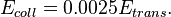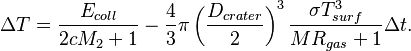# Collision Heating

When two objects collide, some of the kinetic energy of the collision will heat the objects, increasing their temperatures. Universe Sandbox simulates this collisional heating by calculating the expected temperature change based on the parameters of the collision and adjusting the surface temperature properties of each object.

## Models

During a simulated collision, a portion of the mass of the smaller object is transferred to the larger object. Universe Sandbox assumes that a small fraction of the kinetic energy of the transferred mass in a collision, Etrans, is used to heat the colliding bodies:$E_{coll} = 0.0025 E_{trans}.$

The change in a colliding object's temperature, ΔT, is calculated from this incoming energy, Ecoll, which increases the temperature based on the specific heat capacity of rock, c and the mass of the smaller object in the collision, M2. Some of the heat from the collision is lost as the energy is radiated into space from the melted crater. The amount of lost heat depends on the object's mass, M, and surface temperature, Tsurf, the crater diameter, Dcrater, the Stefan-Boltzmann constant, the specific gas constant, Rgas, and the current simulation time step, Δt. The full equation used to calculate the temperature changed based on the incoming and outgoing heat energy is$\Delta T = \frac{E_{coll}}{2cM_2 + 1} - \frac{4}{3}\pi \left(\frac{D_{crater}}{2}\right)^3 \frac{\sigma T_{surf}^3}{M R_{gas} + 1} \Delta t.$